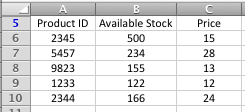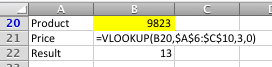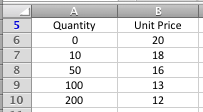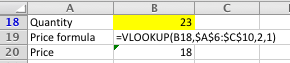# How to use VLOOKUP in Excel

VLOOKUP is a powerful Excel function that allows you to look for a specified value in one column of data inside a table, and then fetch a value from another column in the same row.

An example where VLOOKUP might be useful is if you have a monthly sales report in Excel, and want to find the sales made by a specific salesperson from within a monthly sales report. You would lookup the the person's name in the Salesperson column, and then look in the Sales column to find that person's sales for the month.

In this lesson you'll learn how to use VLOOKUP in your spreadsheets. We'll take you through several simple examples where you can see VLOOKUP in action.

## Scenarios where VLOOKUP is useful

VLOOKUP is useful for the following scenarios - no doubt you can think of examples of your own:

• Calculating the commission for a sales employee, given the value or quantity of sales they have made (look up the salesperson's name, find their sales, and multiply by the commission rate).
• Deciding which commission rate to pay based on the level of sales (look up the actual sales in a commission table to find the appropriate commission percentage to pay).
• Looking up the price of a given product from a table of product information (look up the product name or part number and return the price for that product)
• Looking up the price of a product for a given sales quantity (look up the number of items being ordered and find the price to be charged for that volume).
• Checking the date an employee started work, given the employee's staff ID number (look up the staff ID number and return their start date)..

The VLOOKUP function is particularly useful as an alternative to using multiple nested IF statements, particularly once you have more than two or three nested IF functions in your formula. Formulas with multiple IF statements can get very complicated. Often, a long formula with lots of IF functions can be replaced by a single VLOOKUP formula.

## VLOOKUP() Function Syntax

The VLOOKUP() function has the following syntax:

=VLOOKUP(lookup_value, lookup_table_range, column, exact)

VLOOKUP() works by taking the value you are looking up (lookup_value) and looking for it in the first column of the table you are searching in (lookup_table_range).

Once the function finds the matching value in the lookup_table_range, it then reads across that row the table to the column you chose and returns the value it finds there.

In some cases you want the VLOOKUP function to find the nearest match, and sometimes you'll want the exact match. In this function, exact is an optional value (that's why it's not shown in bold above). if you set the exact parameter to 1 the formula will look for the nearest value. If you set it to 0 (or leave it out), it will look for an exact match and return a #N/A error if it can't find an exact match.

## Examples of the VLOOKUP() function in action - VLOOKUP with an exact match

Here is an example of how the VLOOKUP() function is used to find a value based on an exact match.

• Suppose you have a table that looks like the following:• You've been asked to come up with a way to check the price of a product when a product ID is typed into a given cell
• The formula you type in looks like this:• In the table above, the VLOOKUP function looks for the value found in B20 (9823) in the first column of the range of cells from A6:C10 (i.e. the table in the previous step).
• Having found it in the first column (column A) it then reads across to the third column (column C) and retrieves the value. In this case, the value retrieved is 13.
• In case you're wondering, the \$ signs in the function (\$A\$6:\$C\$10) mean that if you copy the formula in B21 to another location, it will continue to look in the product table. This is an example of absolute references in action; find out more about absolute references here. Note that if your VLOOKUP function generates a #N/A error, it could be because you didn't use absolute references, and then copied and pasted the VLOOKUP formula from one cell to another
• No doubt you're sharp enough to have spotted the 0 as the exact, or fourth, parameter in the function in B21. This means that the VLOOKUP function is looking for an exact match. If it hadn't found the value from B20 (9823) in the first column of the table, the formula in B21 would have returned a #N/A error, meaning Excel couldn't return a value from the function. Remember, of course, that the exact parameter is optional, and defaults to 0 if you don't include it, so in this case we could have left this value out altogether and got the same result.
• If you want to become a VLOOKUP() ninja, here's something important you need to know about the exact parameter in the VLOOKUP function:
• I'm sure you noticed this already, but did you see that the items in the Product ID table are not sorted in any order.
• When using the VLOOKUP function, you need to check if the column you're performing the VLOOKUP on is sorted in ascending order (i.e. smallest values first, which means 1,2,3,4 or A,B,C,D). Sorting them in descending order (largest values first) isn't the same.
• If the first column in your lookup table isn't sorted, you MUST use the exact version of the VLOOKUP function, as we did in this example. This forces the VLOOKUP() function to find an exact match. If an exact match cannot be found, the function will return a #N/A error.
• If we had put 1 instead of 0 in our formula in the example above, the VLOOKUP function would have looked down the list in column A until either it found the value it was looking for, or it found a value that was larger than the value it was looking for, and then taken the previous value in column A as the nearest match. More on "nearest matches" with the VLOOKUP function in the next example.

## Examples of the VLOOKUP() function in action - VLOOKUP with the nearest match

Here is an example of how the VLOOKUP() function is used to find a value based on an exact match.

• Suppose you have a table that looks like the following:• This table represents a scenario where an order between 1 and 9 units will cost 20 per unit. An order between 10 and 49 units will cost 18 per unit, and so on.
• It's important that you understand this before you go onto the next step, otherwise you'll get confused and the result the VLOOKUP function returns.
• Note that in this scenario, any order over 200 units will cost 12 per unit.
• We have also assumed that the minimum order quantity is greater than 0. However, the VLOOKUP function has no problem with using negative numbers if that's what your particular situation requires.
• In this example, you've been asked to come up with a way to check the price of a product for a given sales quantity. The method you choose should work for any sales quantity that is entered.
• You set up a VLOOKUP formula as follows:• The formula works by looking up the value in B18 (23) in the first column of the table (column A) in the previous step.
• Because the VLOOKUP() function is using the nearest match, it goes down column A looking for a match with the value in B18. In this example, it doesn't find one, and eventually it reaches the value in A8 (50) which the first value larger than the value it is looking for (23). At this point the VLOOKUP function stops looking and moves to the next step.
• This is why, for VLOOKUP with a nearest match, you MUST sort the lookup table so the first column is in ascending order. If you don't, VLOOKUP may stop looking too soon, and ignore the possibility that a better (or exact) match existed further down the table.
• The VLOOKUP function then drops back to the previous row (10) and reads across the table to the second column and retrieves the value it finds (18).
• It's worth noting that it is very common when using the VLOOKUP table to get the design of the lookup table wrong. If your VLOOKUP function isn't working properly, check the design of the table first to make sure that it is correct.

As I said earlier, the VLOOKUP() function in Excel is very powerful. These are two examples of how to use it, but there are many more ways it can be applied. If you have a scenario where you think the VLOOKUP() function might apply but you can't figure it out, or you have another situation where you've successfully used the VLOOKUP() function in Excel, why not tell us about it the comments below?

## Our Comment Policy.

Add a comment to this lesson

## Comments on this lesson

### IF and VLOOKUP

I tried to use the IF and the VLOOKUP functions, but I got #VALUE.
The first sheet contains listings of Type, Model and Price. The next sheet has the "type", and looking for the average and count of that particular "type". I'm still trying to figure out the formula, coz I did answer some of the problems. Need help.

Hi Jolly

Thanks for your comment. Can you give me some more information, such as some sample data and the formula you are using? #VALUE errors can arise for a number of different reasons, so more specific information will help me troubleshoot your problem.

Regards

David

UCD?

### I understand what you

I understand what you explained here, but I have a major issue with the VLOOKUP formula. I also tried the LOOKUP, HLOOKUP and others to no avail.

My issue is that the LOOKUP_Value in my case is not in the first column in the table. It is in the 3rd column of the table and the fourth column of the spreadsheet. I need a formula to find the match without sorting or re-ordering my dataset. My formula returns N/A. What do I do then?

### Excel Formula

I need a formula which calculates rates based on no. of copies and the total amount. My total amount shouldnot exceed or should be less than 5000. It should be always 5000. But my number of copies will keep on change. And I need to calculate rate based on this criteria. Please help me.
For example,

sr no Copies Rate Amount
1 1043 X 0
2 100 X 0
3 50 X 0
4 40 X 0
Total 1233 X 5000

### Your article on VLOOKUP was

Your article on VLOOKUP was well done. I had no problem following your explanations and was able to immediately put this function to good use. Thank you!

### Dynamic file ref

Hi,

Hope you can help, I was wondering if there was a way in which I could get the file ref in my formula below to update automatically.

IF(ISERROR(VLOOKUP(N1,'J:\Purchasing\DELIVERY ISSUES\Goodsin issues 2014\[Goodsin issues WE 150214.xlsm]Issues per Supplier'!\$D\$3:\$E\$100,2,FALSE)),0,VLOOKUP(N1,'J:\Purchasing\DELIVERY ISSUES\Goodsin issues 2014\[Goodsin issues WE 150214.xlsm]Issues per Supplier'!\$D\$3:\$E\$100,2,FALSE))

The file 'Goodsin issues WE 150214' changes weekly (as this report is collating data from the previous week). So without having to use the replace function, is it possible that I could just put the date of the file I want to ref in another cell in the spreadsheet and the formula would recongnise this? And therefore retrieve the data from the correct file. (NB: is is only the date part of the file that changes)

Thanks

Dave

### FIND VLOOKUP SECOND MATCH

Please help me how to find second match by vlookup or any other function

### Finding more than one value, when VLOOKUP only finds one

Hi Saji

Check out this lesson if you want to find the second instance of an item in a list. VLOOKUP won't do it, but the INDEX function will (with a bit of coaxing).

//fiveminutelessons.com/learn-microsoft-excel/use-index-lookup-mult...

Regards

David

### Nested If

I have been working on created a nested IF for a document I am working on, however I have maneged to make two diffrent IF statemnets that both work but they need to be merged.

The infomation its in relation to is:

J K L N
Start End Cloud
23 01/04/2014 cloud Yes
24 03/03/2014 Overdue
25 22/05/1992 cloud Yes
26 03/03/2014 Overdue
27 01/07/2014 cloud Yes
28 29/06/2014 On Time
29 03/03/2014 10/03/2014 Complete Yes

The two IF's i current have are:

This If statement firstly looks to see if the End column is filled if it is filled then the project is completed and that can be Displayed in column L. Then it takes the start(date) in ciolumn J and adds 10 workdays it then compares this to todays date to see if the project is Overdue or ontime.

=IF(NOT(ISBLANK(K25)),"Complete",IF(WORKDAY(J25,10)<TODAY(),"Overdue","On Time"))

This IF statement looks to see if there is a Yes in the Cloud collumn(N) if there is it then adds 20 working days to the start date then compares that to todays date to see if the project is overdue or on time.

=IF(AND(N26 = "Yes", (WORKDAY(J26,20)<TODAY())),"Overdue", "On Time")

So what I am looking for is these two combined but i always get an error or a emssage saying to many arguments.

What it needs to do is:
1) look to see if their is and end date if there is set column L to complete
2) see if there is a Yes in the cloud collumn if so add 20 workdays to the start date the compare to todays date and set column L to either overdue or on time
3) if the cloud column is empty add 10 workdays to the start date then compare to todays date and set column L to either overdue or ontime.

Any help would be awesome!!!

### Formula to return values in a row

Hello,

I was wondering if there is a formula that returns values across in a row when a reference value is entered in the first coloumn.

Regards,
RM

I tried to do put formula when I was asked about the excel sheet has 1, 2, 3, 4, 5 etc and I need to insert a column beside and give 1=24, 2=48, 3=72, 4=96, 5=120. Is it possible to do this?

thank you!!

### If or V-Lookup????

How would I get the Ending Balances (F4:F7) to populated according to what is entered in the #LOA hours taken and Type of Absence (C and D columns)?

Beginning balances are filled in E4:E7.

Hope this makes sense.

### Help with Vlookup

I have one worksheet which has a full list of file numbers and then there are other worksheets where these file numbers are split up with further information. Is there a formula that I can use to find the file number from the main worksheet in the other worksheets and for it to list which worksheet it is actually recorded on.
Not sure if you can even get your head around that!

### Lookup Table

Very useful well written article. Thanks

### excel lookup price multiplied by quantity #value

This is the formula: =IF(D25<=0,"",VLOOKUP(D25,PRODUCT_PRICEDB!\$A\$3:\$C\$999,3,FALSE))*(J25)

When all cells are filled it gives the desired result but if one cell is empty i get a #VALUE error. Please help.

### mujy ye samaj nai aye ka last

mujy ye samaj nai aye ka last pr 3,0 likha tha chalo 3 to columns hyn to zero q aya or second example ma 2,1 q aya? plz explain me in vlookup formula

### Referencing

I am currently unable to duplicate values in Consolidated sheet automatically. For example, in Consolidated sheet, column F2, when dragged at its corner should replicate the formula in (F2) to F3 and henceforth.

Right now, the values in F3 and all other columns when dragged, need to be edited for correct column numbers.

### Nice Post

Hi, It seems very useful post, and I learn some advance topic indeed.
I also got a link on vlookup for beginners
https://goo.gl/dLZMGq
I hope they will be able to get some quick idea on excel before moving advance excel

### VLookUp - Identifying the column

Hi. I noticed that you have to input which column you are looking for when you are writing out the formula. Using your example i.e. =VLOOKUP (B20, A6:C10, 3,1), the "3" would have referred to Column C <which essentially is the price>. But what if I am looking at a big sheet where the column is let's say Colum AZ. How would I know AZ is column number what? Do I virtually have to count the columns?

### Excel Head ache

Hie, i have a table like this and i would like to construct a table like this one:
MEMBER COVER AGE PREMIUM

If for instance, the Member is a Principal and his Cover is \$500.00 and Age is 18-25, the formula under Premium must AUTOMATICALLY pick \$7.83.
this can apply to any type of member with their different covers and ages.

Jabu

### You need to use a range lookup.

With this kind of problem, you need to use a range lookup instead of an exact match lookup.

An Exact VLOOKUP will search for the exact age.

Let's say a member age is 19 which doesn't exist in the lookup column, your formula will result in an error should you use an exact match.

So to skirt this problem, you need to use a range look up.

With the range lookup, excel will analyze the lookup value and see which age category it falls.

For more info. about range lookup, check out these VLOOKUP examples: https://softwareaccountant.com/vlookup-example/

Thank you.

### Over time Calculation for Friday.

My company introduced new policy regarding over time. Before that overtime was count as double (2x) for each hour (for 7 days), now for Friday policy is changed. 12:30 to 16:00 its single (1x) after 16:00 hour it'll again double (2x). can some one suggest me any formula which may ease my work. Attached sheet is example